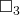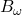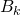# Monthly Archives: February 2020

## Modal Logic Provability

Here’s a much harder reduction based on the modal logic from last time.

The problem: Modal Logic Provability.  This is problem LO14 in the appendix.

The description: Given a modal system S that follows the “S4” modal logic rules, and a modal statement A, can A be proven in S?

Example: S4 modal logic is concerned with what different agents know.  I found a pretty good example of how it works from the notes of a lecture at CMU (Section 2, the story about the hats).

From our point of view, we would say that statement A would beB3. (The third person knows his hat is black), and the explanation of how we got there would be the proof of the statement.

Reduction: Ladner’s paper has the reduction from QBF.  It’s theorem 3.1 in the paper if you want to look at it.  They start with the setwhich is the set ofsets that the Stockmeyer and Meyer paper used in their QBF proof (I talked about it in my discussion of Sequential Truth Assignment), but with any number of quantifiers.  From a QBF formula A, they will build a modal formula B (sort of like the A in our problem definition) with the property that A is a satisfiable QBF formula if and only if B is S4-Satisfiable.

(He actually proves that it for B  “between” K-Satisfiable and S4-Satisfiable.  K-satisfiable is a slightly simpler set of rules.)

The way he builds B is pretty crazy and hard to follow.  I’m not sure there’s much I can say about it that’s not just requoting the entire paper.  The basic idea though is that he is using the modal operators in B to replace the quantifiers in the QBF formula.  The various levels of “for all” and “there exists” are replaced by worlds for agents “know” that a fact is true.

So the clauses in the formula form a tree, and every “For all” in A leads to a branch of worlds (one where the variable is true, and one where it is false).  Then he shows that

Difficulty:8.  Maybe less if you’re comfortable with the Modal logic rules.